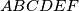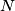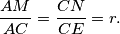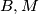### IMO Shortlist 1982 problem 5

Kvaliteta:
Avg: 0.0
Težina:
Avg: 0.0
The diagonals$AC$ and$CE$ of the regular hexagon$ABCDEF$ are divided by inner points$M$ and$N$ respectively, so thatDetermine$r$ if$B,M$ and$N$ are collinear.
Source: Međunarodna matematička olimpijada, shortlist 1982